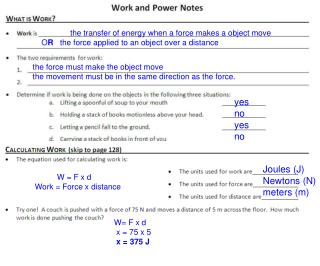DownloadDownload Presentationthe transfer of energy when a force makes a object move O R the force applied to an object over a distance

# the transfer of energy when a force makes a object move O R the force applied to an object over a distance

Download Presentation## the transfer of energy when a force makes a object move O R the force applied to an object over a distance

- - - - - - - - - - - - - - - - - - - - - - - - - - - E N D - - - - - - - - - - - - - - - - - - - - - - - - - - -
##### Presentation Transcript

1. the transfer of energy when a force makes a object move OR the force applied to an object over a distance the force must make the object move the movement must be in the same direction as the force. yes no yes no Joules (J) Newtons (N) meters (m) W = F x d Work = Force x distance W= F x d x = 75 x 5 x = 375 J

2. the rate that work is done OR the amount of work done in 1 second Watts (W) Joules (J) seconds (s) Work time Power = W t W t 50 2.5 x = 20 Watts P = P = x = 50 1.25 x = x = 40 Watts INCREASE!!!